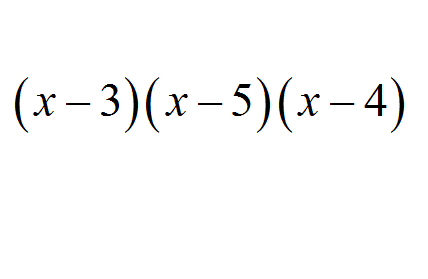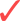# Factor by Grouping

Factor by grouping is an excellent way of factoring an expression, without the need of solving a polynomial equation, which could be hard to solve.

The only problem of factoring by grouping is that there is not one recipe or strategy that will give you the proper grouping that is needed. Or even worse, there may not be a clear way of grouping in order to conduct a factorization.In this tutorial we will concentrate on the special cases in which grouping will help to factor an algebraic expression, although the truth is, it is not always possibly to do. For a more general treatment, check out this tutorial on how to factor .

## The Conditions Required for Factoring by Grouping

This is how factoring by grouping works:

We need to look for certain hints in order to use this kind of factoring. For starters, we will expect to have an algebraic expression with an even number of terms that is greater than 2 (so 4, 6, etc), and then try to group.

Like we said, there are no fixed rules, and you need to play it by ear, following these two steps.Step 1: Group the first and second term, third and fourth term, and so on.Step 2: Now, try to factor all the pairs that you grouped in Step 1. Observe that there could be more than one way to factor.Step 3: See if the factors you obtained in Step 2 are all the same, in which case, you can factor it.Step 4: If the previous steps do not work, try the trick of "adding zero": Sometimes, things will work out if you add something, and you also subtract it from the expression.

By adding and subtracting the same term, the net effect is the same as adding (which is, leaving the expression the same as it was)

### EXAMPLE 1

Factor using the method of Factor by Grouping the following polynomial

$6x^3 + 3x^2 - 4x -2$

We need to use the steps that we defined above. Notice the these steps are not set in stone, but they are a useful guidance for you to follow:

Step 1: We group the first and second term, and also the third and fourth term so we get

$6x^3 + 3x^2 - 4x -2 = (6x^3 + 3x^2) - (4x + 2)$

Step 2: The term $$6x^3 + 3x^2$$ is factored as $$6x^3 + 3x^2 = 3x^2(2x+1)$$, and the term $$4x + 2$$ is factored as $$4x + 2 = 2(2x+1)$$, so we get:

$6x^3 + 3x^2 - 4x -2 = (6x^3 + 3x^2) - (4x + 2) = 3x^2(2x+1) - 2(2x+1)$

Step 3: Now we can see how the two groups that we factored have a common factor, which is $$2x+1$$, which can be factored out by the distributive property. Therefore, the following is obtained:

$6x^3 + 3x^2 - 4x -2 = (3x^2-2)(2x+1)$

which concludes the factoring process.

### EXAMPLE 2

Solve the following equation: $$x^3 -6x^2 + 11x - 6 = 0$$:

Since we don't really know (although it is possible) how to find the solution of that cubic equation, we need to again use the steps for finding the factoring by grouping of $$x^3 -6x^2 + 11x - 6$$ if possible:

Step 1: We group the first and second term, and also the third and fourth term so we get

$x^3 -6x^2 + 11x - 6 = (x^3 -6x^2) + (11x - 6)$

Step 2: The term $$x^3 -6x^2$$ is factored as $$x^3 -6x^2 = x^2(x-6)$$, and the term $$11x - 6$$ is factored as $$11x - 6= 11(x - 6/11)$$, so we get:

$x^3 -6x^2 + 11x - 6 = (x^3 -6x^2) + (11x - 6) = x^2(x-6) + 11(x - 6/11)$

Step 3: In this case, there is no common factor, so the method has not worked up to this point.

Step 4: We add $$0 = 2x - 2x$$ and add $$0 = 3x^2 - 3x^2$$ which will not affect the expression (we are adding zeros), so we get:

$x^3 -6x^2 + 11x - 6 = x^3 -6x^2 + 11x - 6 + 2x - 2x + 3x^2 - 3x^2$ $= x^3 - 3x^2 -3x^2 + 9x +2x- 6$ $= (x^3 - 3x^2) -(3x^2 - 9x) +(2x- 6)$ $= x^2(x - 3) -3x(x-3) +2(x- 3)$

and now we have the common factor, $$x-3$$ we were looking for. Finally, factoring $$x-3$$ out we get

$\Large x^3 -6x^2 + 11x - 6 = (x^2-3x +2)(x- 3)$

So then, in order to solve the original equation, we can also solve $$(x^2-3x +2)(x- 3) = 0$$ which means that $$x^2-3x +2 = 0$$ or $$x - 3$$ = 0.

From the second equation we have the one solution is $$x = 3$$. From the first equation we need to solve:

$x^2-3x +2 = 0 \Rightarrow x = \frac{-b \pm \sqrt{b^2 - 4ac}}{2a}$ $\Rightarrow x = \frac{3 \pm \sqrt{3^2 - 4(1)(2)}}{2(1)}$ $\Rightarrow x = \frac{3 \pm \sqrt{9-8}}{2}$ $\Rightarrow x = \frac{3 \pm 1}{2}$

which implies that the other solutions are $$x = (3-1)/2 = 1$$ and $$x = (3+1)/2 = 2$$.

## Why Factoring by Grouping?

Let us recall that factoring is always a good thing to solve equation, because when a multiplication of several factors is equal to zero, then the solutions of the equation are found by setting each factor equal to zero.

For example, say you want to solve the equation $$x^3 + x^2 + 2x + 2 = 0$$. I would bet you that you would be clueless if you needed to solve it using algebraic means.

Why? Because this is a cubic equation, and solving a cubic equation is difficult. There is a formula, but it is not an easy one. What alternatives do we have?

Well, we can factor by grouping, if possible. We will see that it is indeed possible in this case. We will follow the steps that were delineated above:

Step 1: Grouping the first and second term, and also the third and fourth term leads to:

$(x^3 + x^2) + (2x + 2) = 0$

Step 2: The term $$x^3 + x^2$$ is factored as $$x^3 + x^2 = x^2(x+1)$$, and the term $$2x + 2$$ is factored as $$2x + 2 = 2(x+1)$$, so we get:

$x^2(x + 1) + 2(x + 1) = 0$

Step 3: Now we see that the two groups we factored have a common factor, which is $$x+1$$, which can be factored out by the distributive property, so we get:

$(x^2+2)(x + 1)= 0$

Therefore, what we have found is that the original cubic expression has been factored as:

$x^3 + x^2 + 2x + 2 = (x^2+2)(x + 1) = 0$

This way, we can solve the equation easily, by setting $$x^2 + 2 = 0$$ or $$x + 1 = 0$$. Notice that since $$x^2$$ is always non-negative we get that $$x^2 + 2 \ge 2$$ and it can never be zero (at least for $$x$$ real).

Therefore the only solution is $$x = -1$$.

So that came for free, using factor by grouping. Otherwise, we would have needed to use a cumbersome cubic root formula, or you would have use the method of "guessing the roots", which let's be honest, it is not really a method.